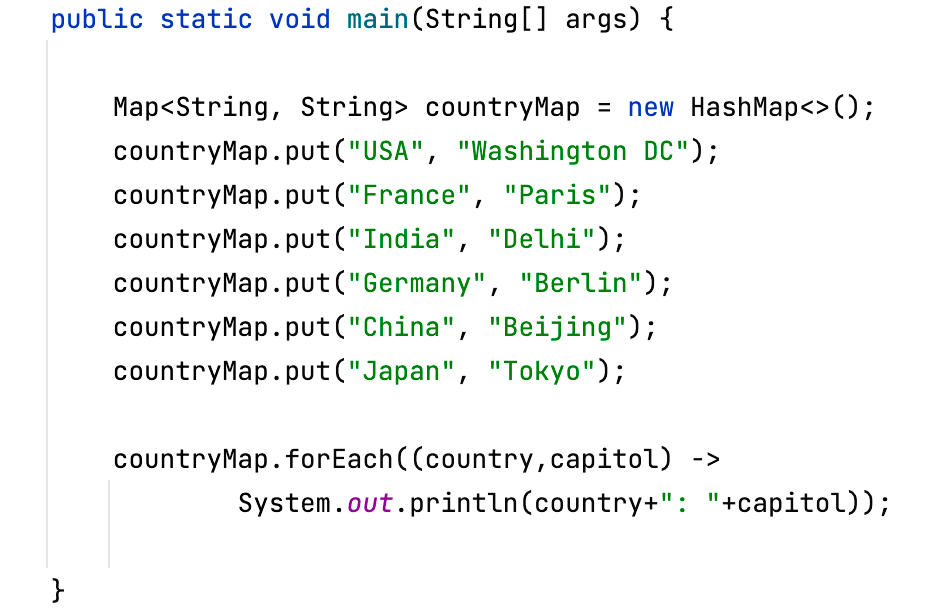# 9 Ways to Loop Java Map (HashMap) with Code ExamplesTo loop a Map (HashMap) in Java we can follow one of the below ways,

1. Using Iterator,
2. Using EntrySet,
3. Using KeySet,
4. Using Java 8 forEach loop,
5. Using Java 8 Stream (and Parallel Stream)

### Example 1: Using legecay for loop and Iterator

``````public static void main(String[] args) {

Map<String, String> countryMap = new HashMap<>();
countryMap.put("USA", "Washington DC");
countryMap.put("France", "Paris");
countryMap.put("India", "Delhi");
countryMap.put("Germany", "Berlin");
countryMap.put("China", "Beijing");
countryMap.put("Japan", "Tokyo");

Iterator<Map.Entry<String, String>> mapIterator = countryMap.entrySet().iterator();

for (int i = 1; mapIterator.hasNext(); i++) {
Map.Entry<String, String> element = mapIterator.next();
System.out.println(i + " - " + element.getKey() + " : " + element.getValue());
}
}``````
Output:
``````1 - USA : Washington DC
2 - China: Beijing
3 - Japan: Tokyo
4 - France: Paris
5 - Germany: Berlin
6 - India: Delhi``````

### Example 2: Using While loop and Iterator,

``````Iterator<Map.Entry<String, String>> mapIterator = countryMap.entrySet().iterator();

while (mapIterator.hasNext()) {
Map.Entry<String, String> element = mapIterator.next();
System.out.println(element.getKey() + " : " + element.getValue());
}``````

### Example 3: Using foreach loop and Map.Entry,

``````for (Map.Entry<String, String> element : countryMap.entrySet()) {
System.out.println(element.getKey() + " : " + element.getValue());
}``````

### Example 4: Using the default foreach method as loop from Map Interface Java 8

``````countryMap.forEach((country,capitol) ->
System.out.println(country+": "+capitol));``````

### Example 5: Using keySet and foreach loop

``````for(String key : countryMap.keySet()) {
System.out.println(key +": "+countryMap.get(key));
}``````

### Example 6: Using keySet, Iterator and While loop

``````Iterator<String> mapIterator = countryMap.keySet().iterator();
while(mapIterator.hasNext()) {
String country = mapIterator.next();
System.out.println(country +": "+countryMap.get(country));
}``````

### Example 7: Using keySet, Iterator and for loop

``````Iterator<String> mapIterator = countryMap.keySet().iterator();
for(int i = 1;mapIterator.hasNext();i++) {
String country = mapIterator.next();
System.out.println(country +": "+countryMap.get(country));
}``````

### Example 8: Using Java 8 Stream API

``````countryMap.entrySet().stream().forEach((element) ->
System.out.println(element.getKey()+": "+element.getValue()));``````

### Example 9: Using Java 8 Parallel Stream

``````countryMap.entrySet().stream().parallel().forEach((element) ->
System.out.println(element.getKey()+": "+element.getValue()));``````